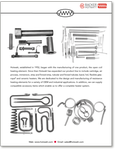Tubular heaters FORMING OPTIONS

Custom formations to your requirements are available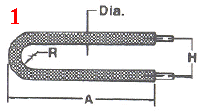SL = 2A + 1.14 R – .43 Dia.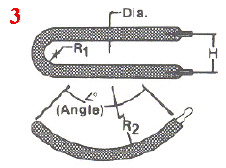SL = .0175<º(2R2 + Dia.) + 1.14R1 – .43 Dia.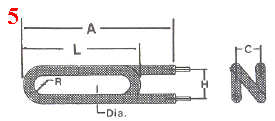SL = 2A + 3.42 R – 1.29 Dia. + 2L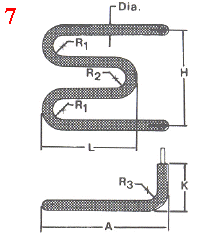SL = 2K – .86 R3 – 3.72 Dia. + 2A + 2L + 2.28 R1 + 1.14 R2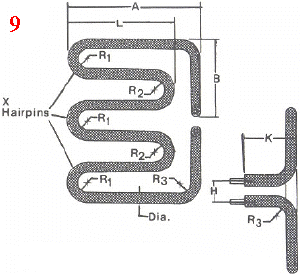X = Number of outhside hairpins
SL = 2K + 2A – 1.72 R3 + 3.14 R1 X + 3.14 R2 (X – 1) + 2L (X – 1) – H + 1.14 X Dia. – 3.42 Dia.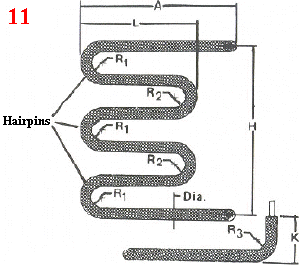X = Number of outside hairpins
SL = 2K + 2A + 2B – 2.58 R3 + 3.14 R1 X + 3.14 R2 (X – 1) + 2L (X – 1) -H + 1.14 x Dia. – 5.85 Dia.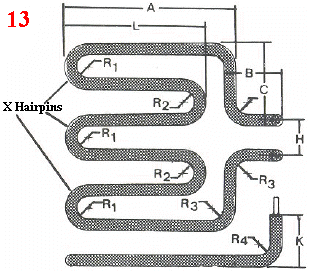X = Number of outside hairpins
SL = 2K + 2A + 2B – 2.58 R3 + 3.14 R1 X + 3.14 R2 (X – 1) + 2L (X – 1) -H + 1.14 x Dia. – 5.85 Dia.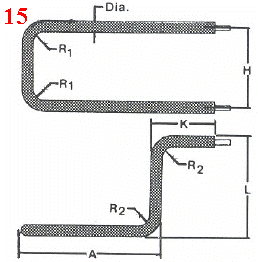SL = 2K – 1.72 R2 – 6.29 Dia. + 2L + 2A – .86 R1 + H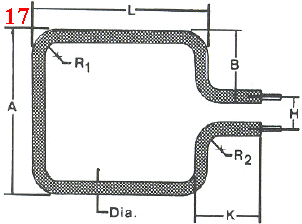SL = 2K + 2A + 2L – H – 1.72 R1 – .86 R2 – 6.29 Dia.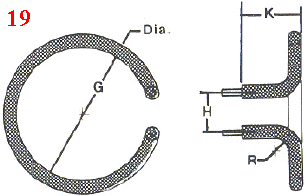SL = (G + Dia.) (3.14) + 1.14 R + 2K + 3.28 Dia. – H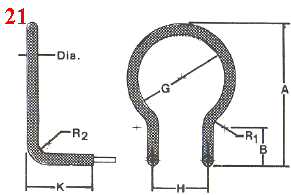SL= (G + Dia.) (3.14)+ 1.14 R1 + 2B + 1.14 R2 + 2K + 3.28 Dia -H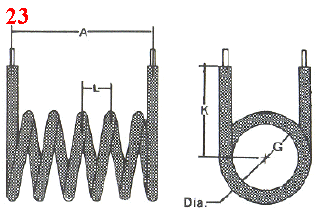SL = [(G + Dia.)(3.14)(Number of 360°’s)] + 2K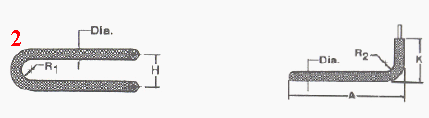SL = 2K – .86 R2 – 2.86 Dia. + 2A + 1.14 R1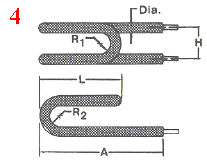SL = 2A + 2.28 R2 – 1.29 Dia. + 2L + 1.14 R1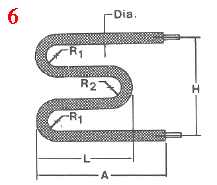SL = 2A + 2.28 R1 – 1.29 Dia. + 2L + 1.14 R2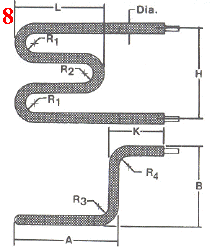SL = 2K – 1.72 R3 – 6.15 Dia. + 2B + 2A + 2L + 2.28 R1 + 1.14 R2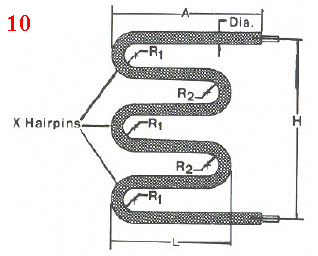X = Number of outside hairpins
SL = 2A + .43 Dia. (1 – 2X) + 2L (X – 1) + 1.14 X R1 + 1.14 R2 (X – 1)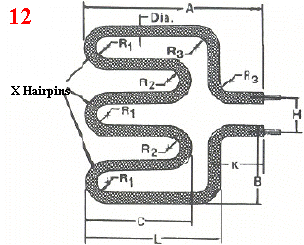SL = 2K + 4C + 2B + 2A + 2L – H – 2.58 R1 – .86 R2 – 12.15 Dia.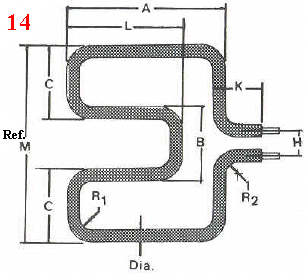SL = 2K + 4C + 2B + 2A + 2L – H – 2.58 R1 – .86 R2 – 12.15 Dia.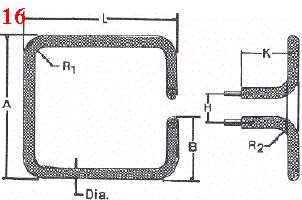SL= 2K + 2A + 2L -H – 1.72 R1 – .86 R2 – 6.29 Dia.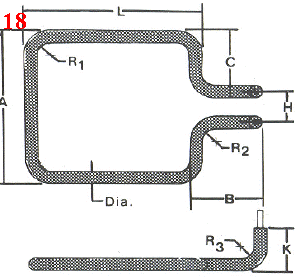SL= 2K + 2A + 2L + 2B – H – 1.72 R1 – 1.72 R2 – 8.72 Dia.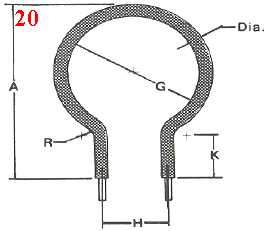SL = (G + Dia.) (.14) + 1.14 R + 2K + 3.71 Dia. – H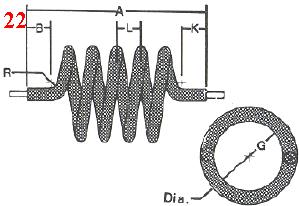SL = [(G + Dia.)(3.14)(Number of 360°’s)] + B + K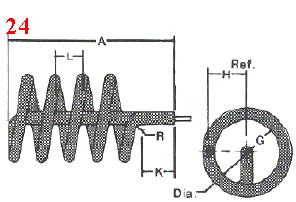SL = [(G + Dia.)(3.13)(Number of 360°’s)] + (G ÷ 2) + A +K